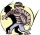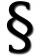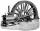Deposit

If you deposit 959 euros the beginning of each year, how much money we have at 2.1% (compound) interest after 19 years?

Result

x =  22111.28 Eur

Solution:Leave us a comment of example and its solution (i.e. if it is still somewhat unclear...):Be the first to comment!Next similar examples:

1. Account operationsMy savings of php 90,000 in a bank earns 6% interest in a year. If i will deposit additional php 10,000 at the end of 6 months, how much money will be left if i withdraw php 25,000 after a year?
2. BankPaul put 10000 in the bank for 6 years. Calculate how much you will have in the bank if he not pick earned interest or change deposit conditions. The annual interest rate is 3.5%, and the tax on interest is 10%.
3. ShuyenShuyen wanted to save some money. She deposited Php.300 in a bank which pays 0.5% interest per annum. After nine months, she needed the money to buy some gifts. How much will she be able to get if he withdraws all her money from the bank?
4. Compound interestCompound interest: Clara deposited CZK 100,000 in the bank with an annual interest rate of 1.5%. Both money and interest remain deposited in the bank. How many CZK will be in the bank after 3 years?
5. Investment1000\$ is invested at 10% compound interest. What factor is the capital multiplied by each year? How much will be there after n=12 years?
6. The crimeThe crime rate of a certain city is increasing by exactly 7% each year. If there were 600 crimes in the year 1990 and the crime rate remains constant each year, determine the approximate number of crimes in the year 2025.
7. JUDr. UsuryJudge JUDr. Usury approved the agreement on guilt and punishment where confessed to pay daily interest 0.18%. How big is a yearly interest? Year has 360 days.
8. MachinePrice of the new machine is € 62000. Every year is depreciated 15% of residual value. What will be the value of the machine after 3 years?
9. PopulationWhat is the population of the city with 3% annual growth, if in 10 years the city will have 60,000 residents?
10. GP - 8 itemsDetermine the first eight members of a geometric progression if a9=512, q=2
11. Six termsFind the first six terms of the sequence a1 = -3, an = 2 * an-1
12. A perineumA perineum string is 10% shorter than its original string. The first string is 24, what is the 9th string or term?
13. Geometric progression 2There is geometric sequence with a1=5.7 and quotient q=-2.5. Calculate a17.
14. Five membersWrite first 5 members geometric sequence and determine whether it is increasing or decreasing: a1 = 3 q = -2
15. Geometric sequence 4It is given geometric sequence a3 = 7 and a12 = 3. Calculate s23 (= sum of the first 23 members of the sequence).
16. Theorem proveWe want to prove the sentence: If the natural number n is divisible by six, then n is divisible by three. From what assumption we started?
17. Tenth memberCalculate the tenth member of geometric sequence when given: a1=1/2 and q=2